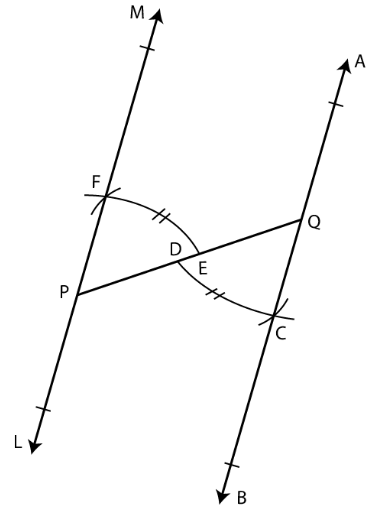# Selina Solutions Concise Maths Class 7 Chapter 14: Lines and Angles (Including Construction of Angles) Exercise 14C

Selina Solutions Concise Maths Class 7 Chapter 14 Lines and Angles (Including Construction of Angles) Exercise 14C provides students with the method of solving problems effortlessly. In this exercise, students can understand the steps to be followed in the construction of angles and special constructions. The problems are solved in a stepwise manner to reduce the fear of solving problems in the annual exam. Here, the students can obtain Selina Solutions Concise Maths Class 7 Chapter 14 Lines and Angles (Including Construction of Angles) Exercise 14C free PDF, from the links which are given here.

## Selina Solutions Concise Maths Class 7 Chapter 14: Lines and Angles (Including Construction of Angles) Exercise 14C Download PDF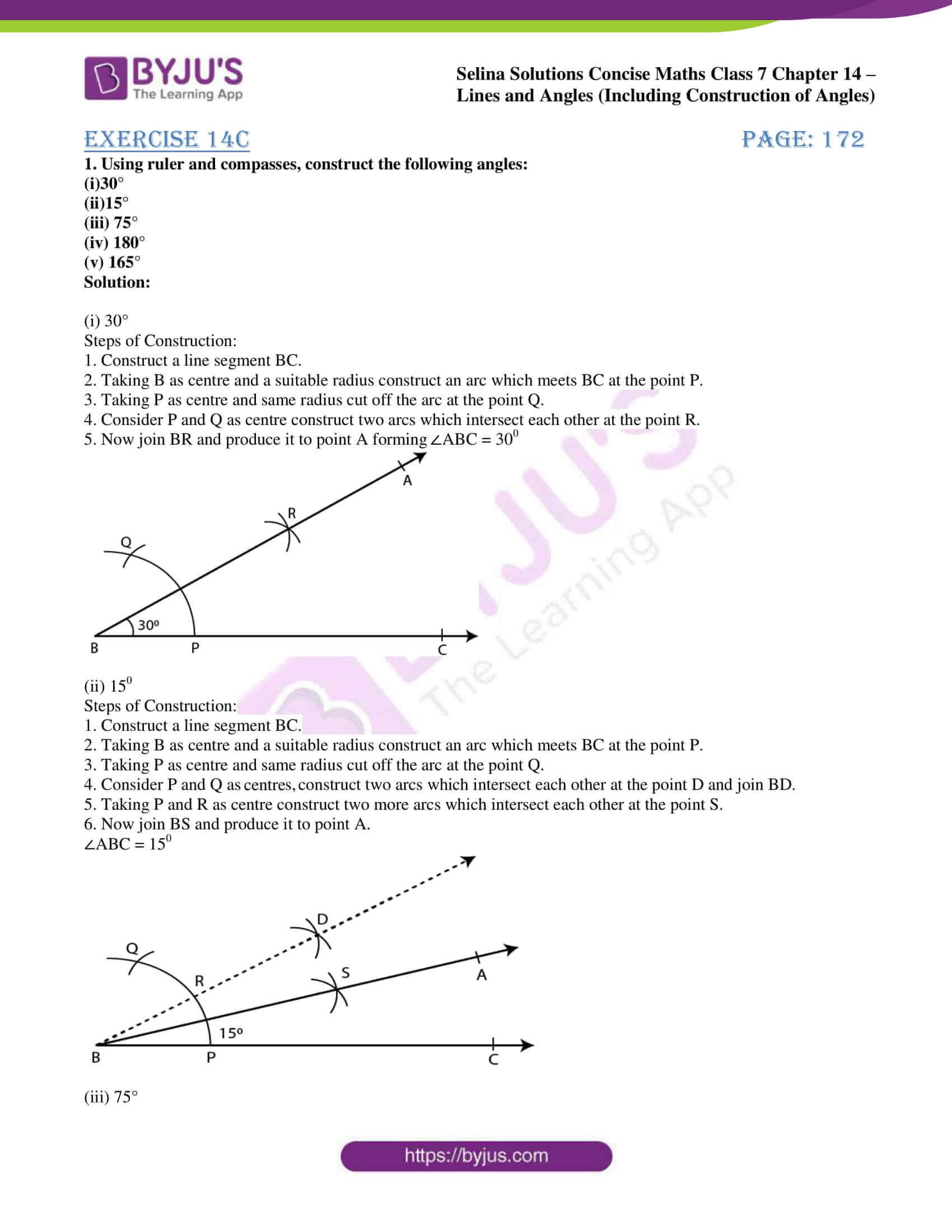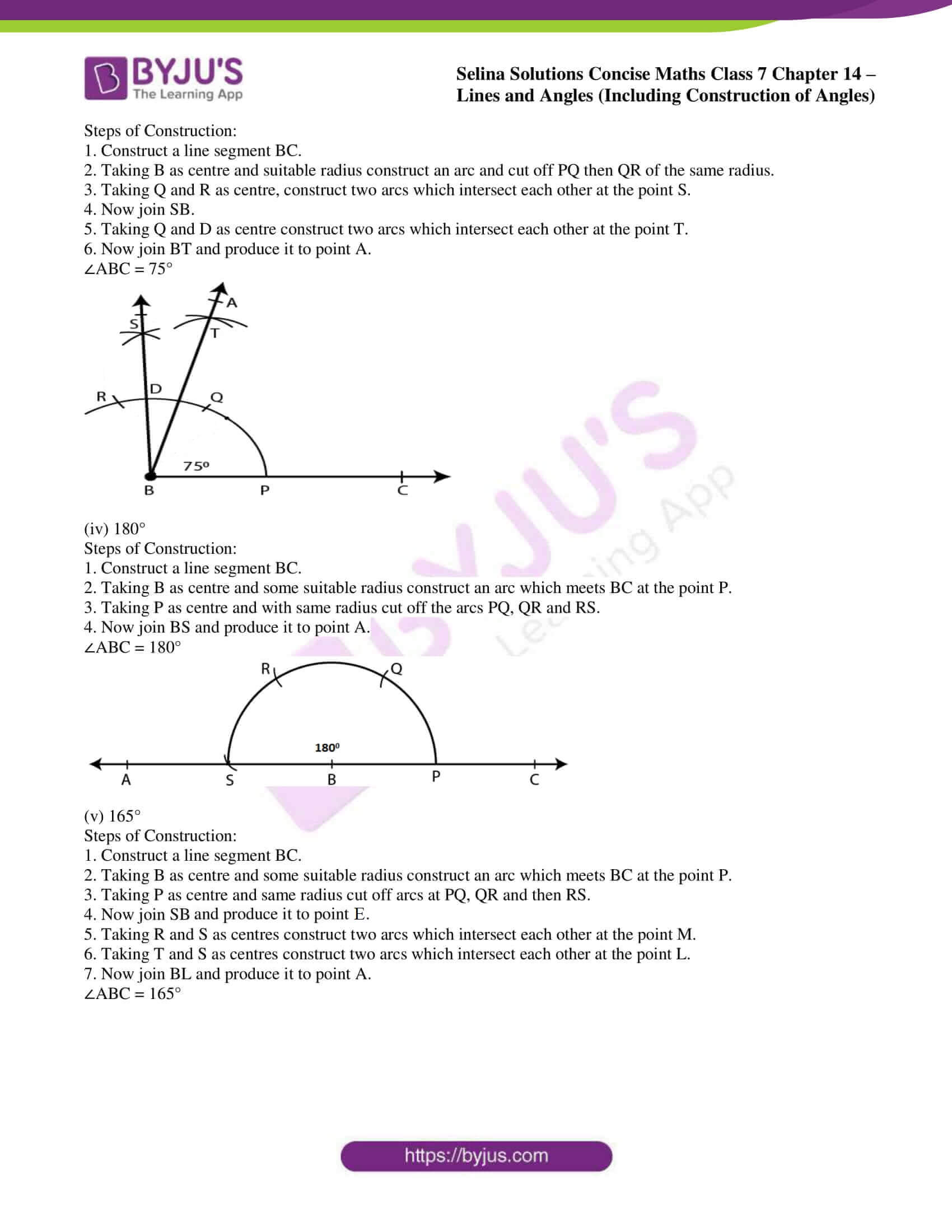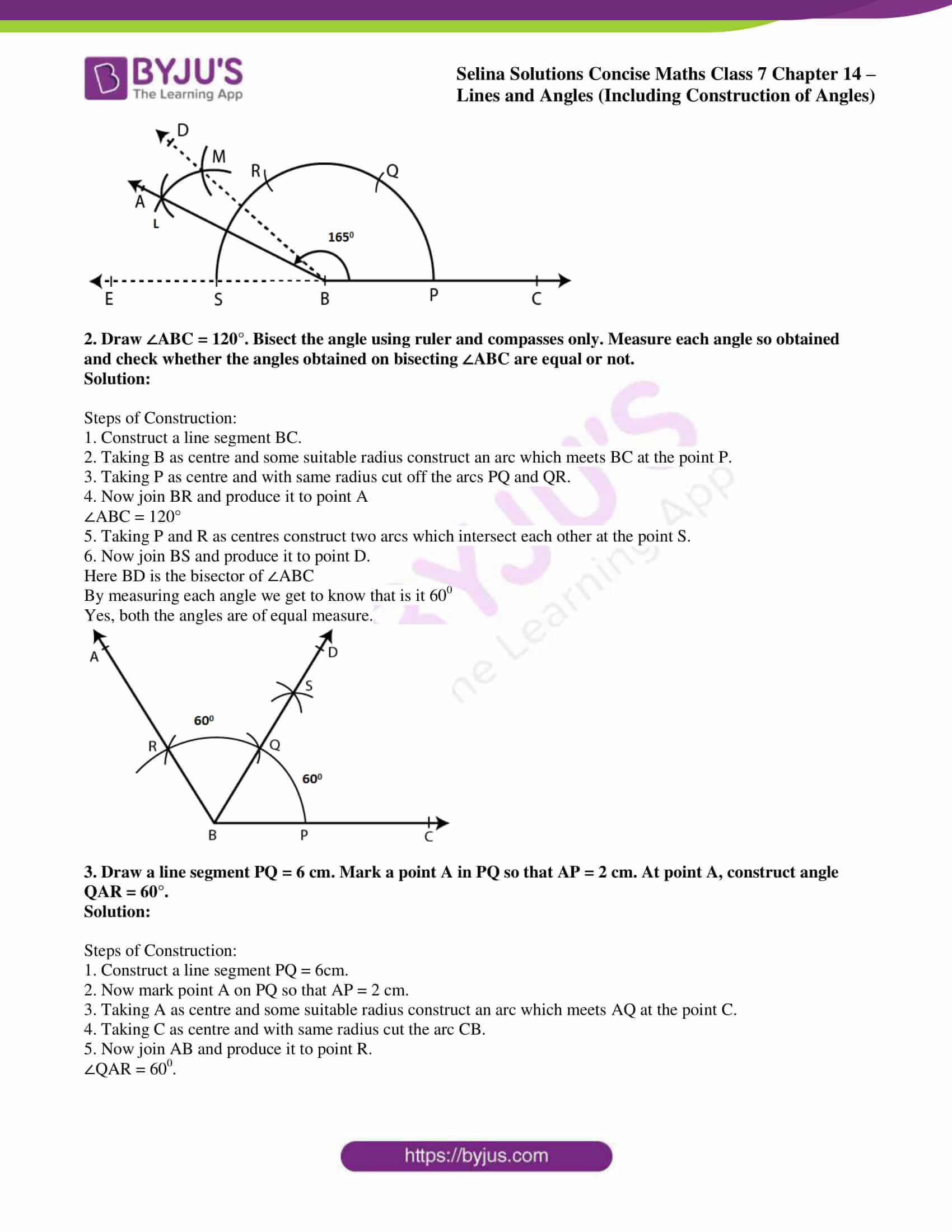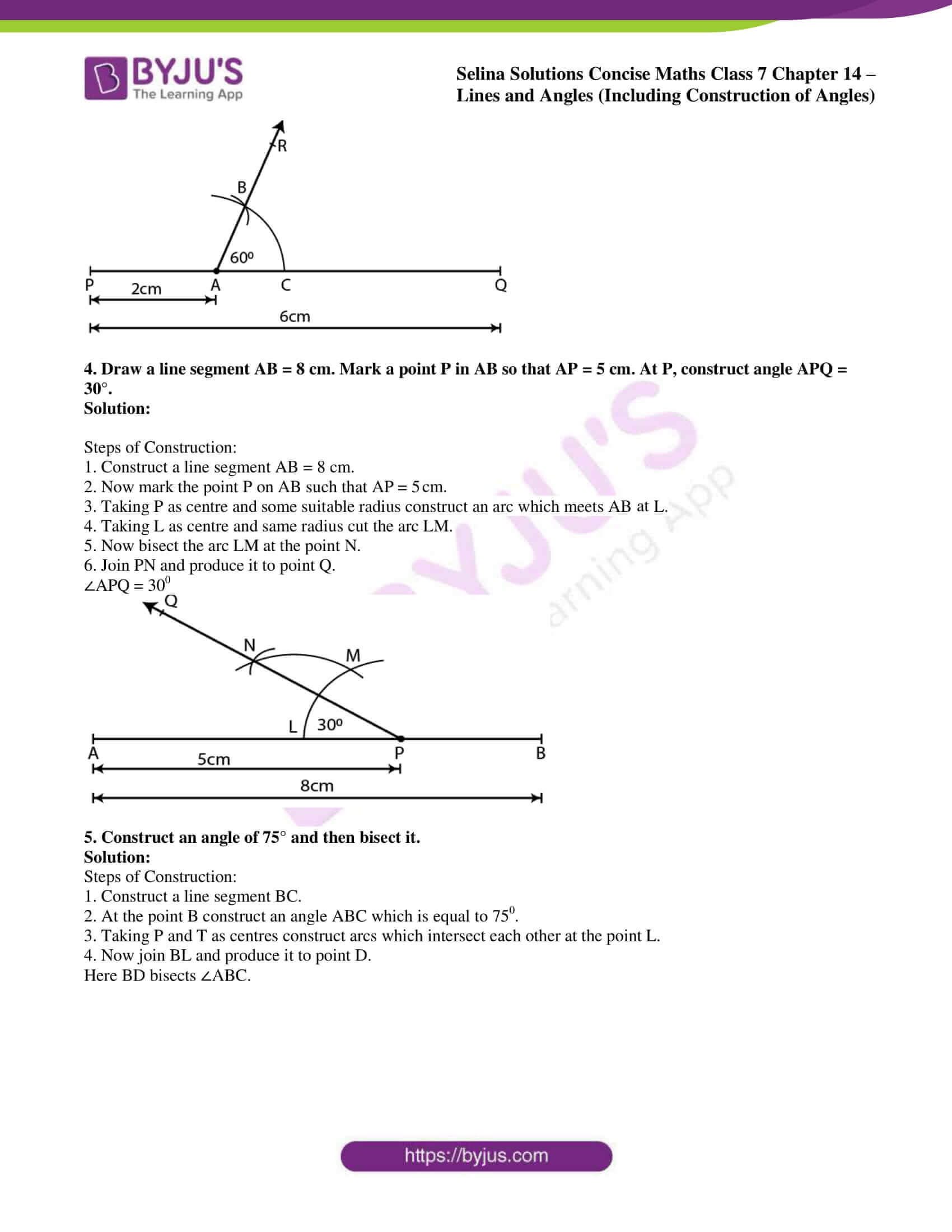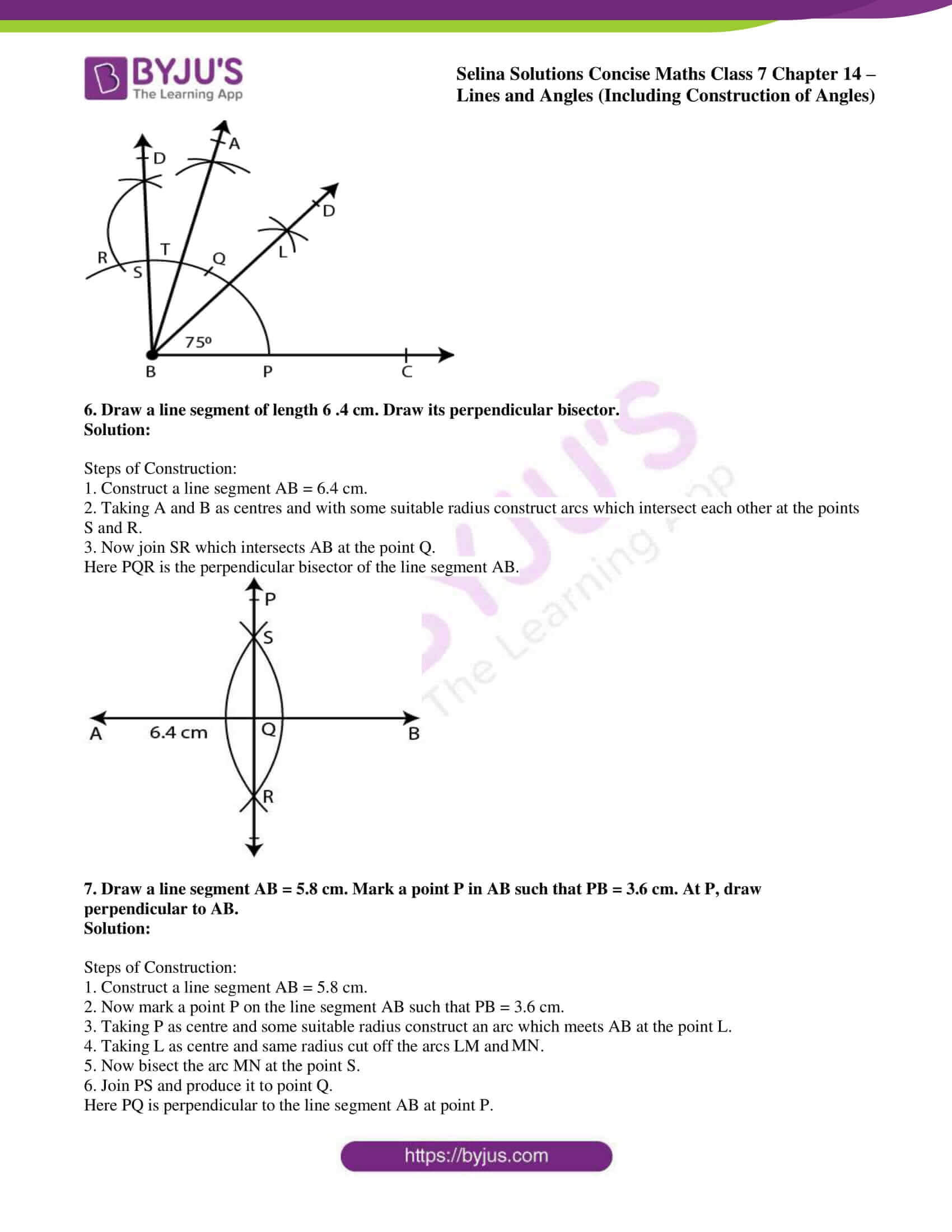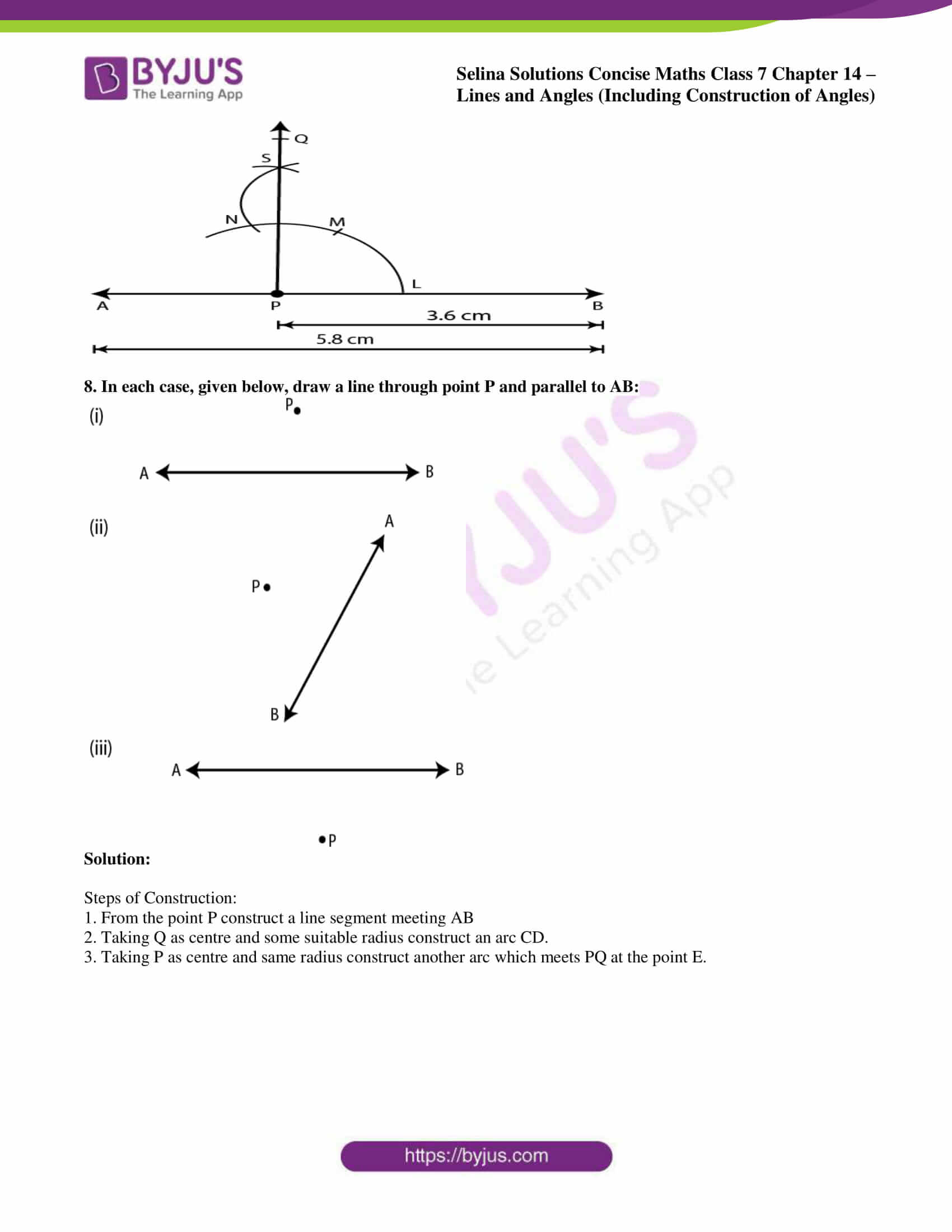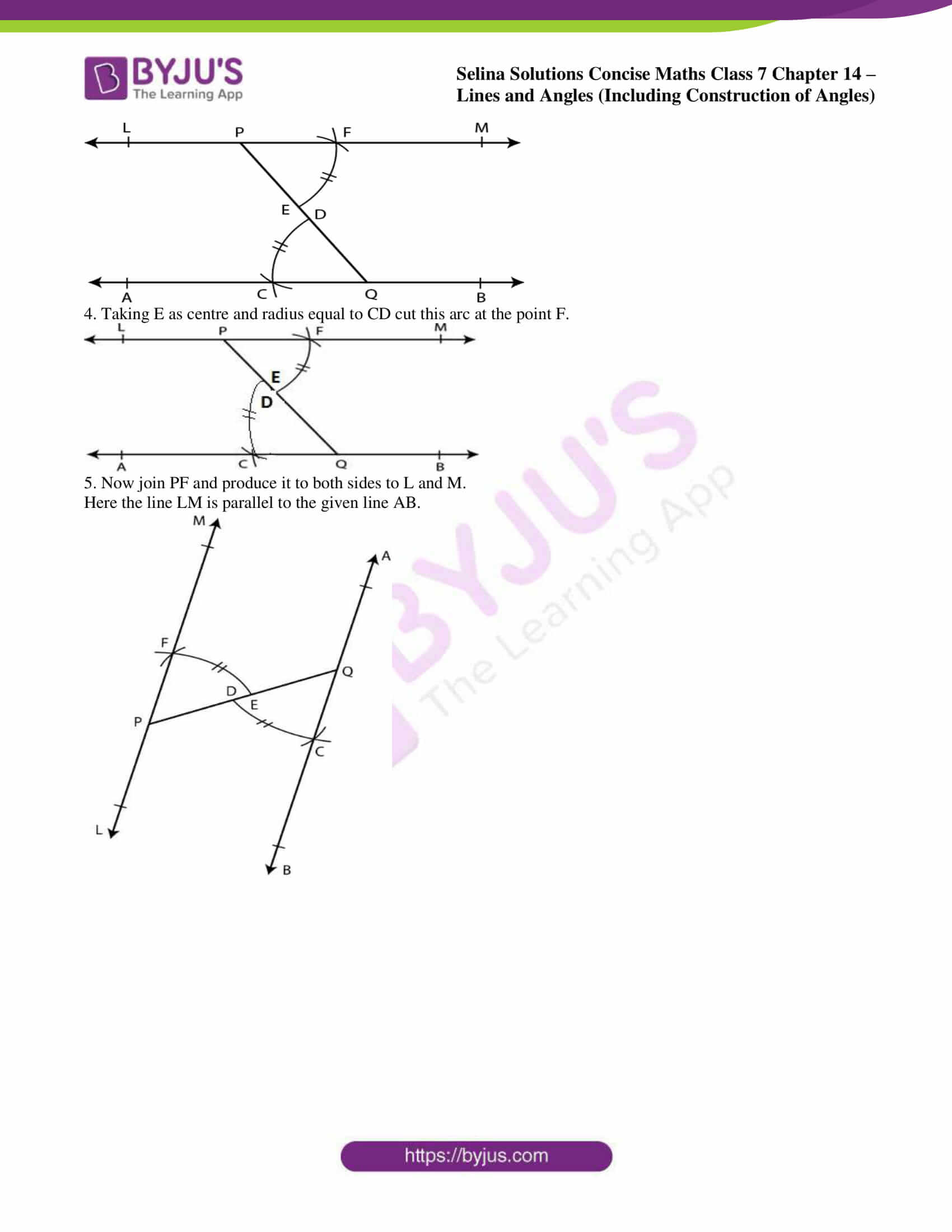### Access other exercises of Selina Solutions Concise Maths Class 7 Chapter 14: Lines and Angles (Including Construction of Angles)

Exercise 14A Solutions

Exercise 14B Solutions

### Access Selina Solutions Concise Maths Class 7 Chapter 14: Lines and Angles (Including Construction of Angles) Exercise 14C

#### Exercise 14C PAGE: 172

1. Using ruler and compasses, construct the following angles:
(i)30°
(ii)15°
(iii) 75°
(iv) 180°
(v) 165°
Solution:

(i) 30°

Steps of Construction:

1. Construct a line segment BC.

2. Taking B as centre and a suitable radius construct an arc which meets BC at the point P.

3. Taking P as centre and same radius cut off the arc at the point Q.

4. Consider P and Q as centre construct two arcs which intersect each other at the point R.

5. Now join BR and produce it to point A forming ∠ABC = 300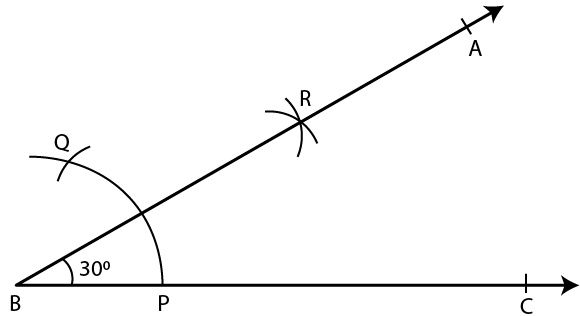(ii) 150

Steps of Construction:

1. Construct a line segment BC.

2. Taking B as centre and a suitable radius construct an arc which meets BC at the point P.

3. Taking P as centre and same radius cut off the arc at the point Q.

4. Consider P and Q as centres, construct two arcs which intersect each other at the point D and join BD.

5. Taking P and R as centre construct two more arcs which intersect each other at the point S.

6. Now join BS and produce it to point A.

∠ABC = 150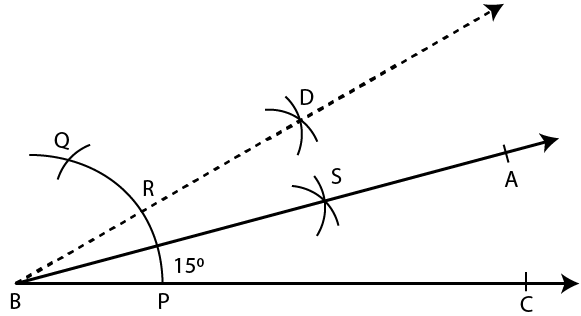(iii) 75°

Steps of Construction:

1. Construct a line segment BC.

2. Taking B as centre and suitable radius construct an arc and cut off PQ then QR of the same radius.

3. Taking Q and R as centre, construct two arcs which intersect each other at the point S.

4. Now join SB.

5. Taking Q and D as centre construct two arcs which intersect each other at the point T.

6. Now join BT and produce it to point A.

∠ABC = 75°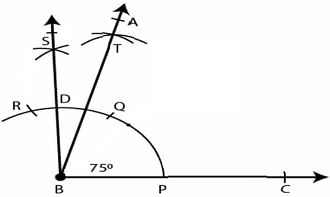(iv) 180°

Steps of Construction:

1. Construct a line segment BC.

2. Taking B as centre and some suitable radius construct an arc which meets BC at the point P.

3. Taking P as centre and with same radius cut off the arcs PQ, QR and RS.

4. Now join BS and produce it to point A.

∠ABC = 180°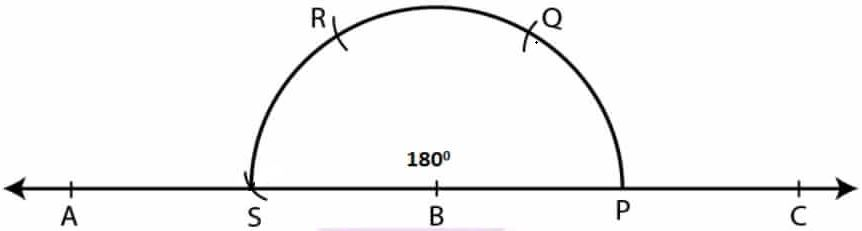(v) 165°

Steps of Construction:

1. Construct a line segment BC.

2. Taking B as centre and some suitable radius construct an arc which meets BC at the point P.

3. Taking P as centre and same radius cut off arcs at PQ, QR and then RS.

4. Now join SB and produce it to point E.

5. Taking R and S as centres construct two arcs which intersect each other at the point M.

6. Taking T and S as centres construct two arcs which intersect each other at the point L.

7. Now join BL and produce it to point A.

∠ABC = 165°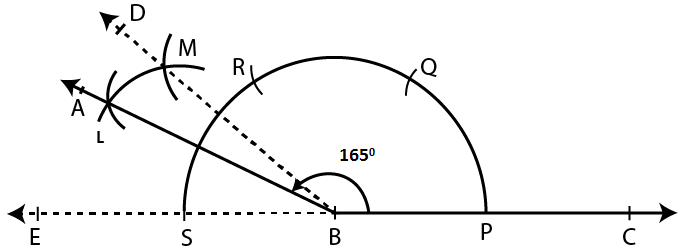2. Draw ∠ABC = 120°. Bisect the angle using ruler and compasses only. Measure each angle so obtained and check whether the angles obtained on bisecting ∠ABC are equal or not.

Solution:

Steps of Construction:

1. Construct a line segment BC.

2. Taking B as centre and some suitable radius construct an arc which meets BC at the point P.

3. Taking P as centre and with same radius cut off the arcs PQ and QR.

4. Now join BR and produce it to point A

∠ABC = 120°

5. Taking P and R as centres construct two arcs which intersect each other at the point S.

6. Now join BS and produce it to point D.

Here BD is the bisector of ∠ABC

By measuring each angle we get to know that is it 600

Yes, both the angles are of equal measure.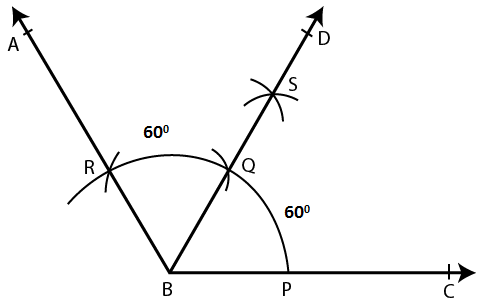3. Draw a line segment PQ = 6 cm. Mark a point A in PQ so that AP = 2 cm. At point A, construct angle QAR = 60°.

Solution:

Steps of Construction:

1. Construct a line segment PQ = 6cm.

2. Now mark point A on PQ so that AP = 2 cm.

3. Taking A as centre and some suitable radius construct an arc which meets AQ at the point C.

4. Taking C as centre and with same radius cut the arc CB.

5. Now join AB and produce it to point R.

∠QAR = 600.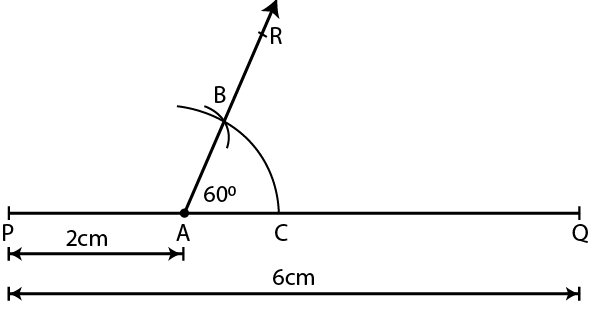4. Draw a line segment AB = 8 cm. Mark a point P in AB so that AP = 5 cm. At P, construct angle APQ = 30°.

Solution:

Steps of Construction:

1. Construct a line segment AB = 8 cm.

2. Now mark the point P on AB such that AP = 5 cm.

3. Taking P as centre and some suitable radius construct an arc which meets AB at L.

4. Taking L as centre and same radius cut the arc LM.

5. Now bisect the arc LM at the point N.

6. Join PN and produce it to point Q.

∠APQ = 300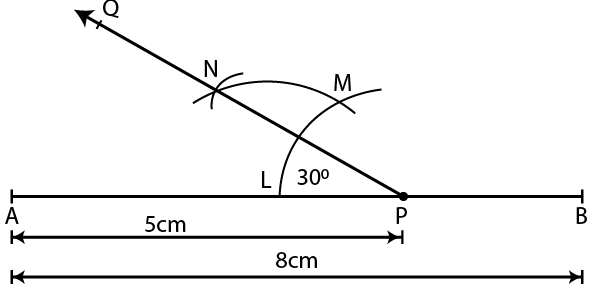5. Construct an angle of 75° and then bisect it.

Solution:

Steps of Construction:

1. Construct a line segment BC.

2. At the point B construct an angle ABC which is equal to 750.

3. Taking P and T as centres construct arcs which intersect each other at the point L.

4. Now join BL and produce it to point D.

Here BD bisects ∠ABC.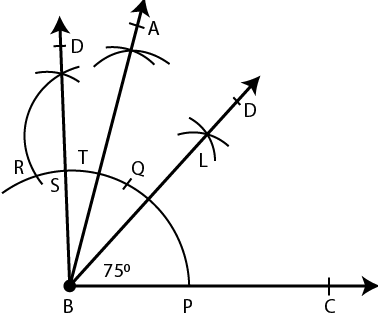6. Draw a line segment of length 6 .4 cm. Draw its perpendicular bisector.

Solution:

Steps of Construction:

1. Construct a line segment AB = 6.4 cm.

2. Taking A and B as centres and with some suitable radius construct arcs which intersect each other at the points S and R.

3. Now join SR which intersects AB at the point Q.

Here PQR is the perpendicular bisector of the line segment AB.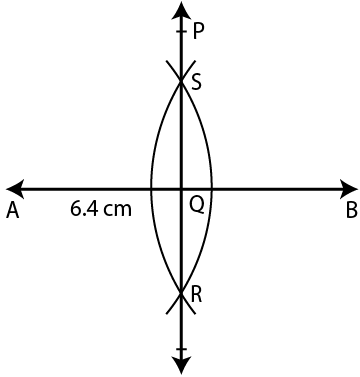7. Draw a line segment AB = 5.8 cm. Mark a point P in AB such that PB = 3.6 cm. At P, draw perpendicular to AB.

Solution:

Steps of Construction:

1. Construct a line segment AB = 5.8 cm.

2. Now mark a point P on the line segment AB such that PB = 3.6 cm.

3. Taking P as centre and some suitable radius construct an arc which meets AB at the point L.

4. Taking L as centre and same radius cut off the arcs LM and  MN.

5. Now bisect the arc MN at the point S.

6. Join PS and produce it to point Q.

Here PQ is perpendicular to the line segment AB at point P.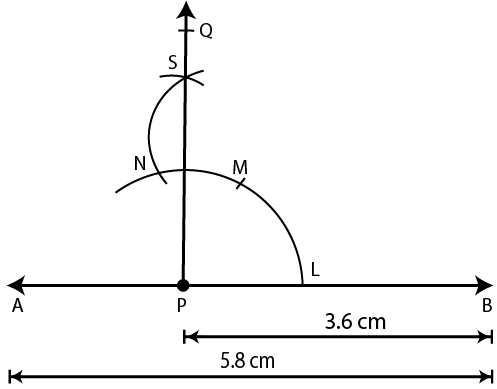8. In each case, given below, draw a line through point P and parallel to AB: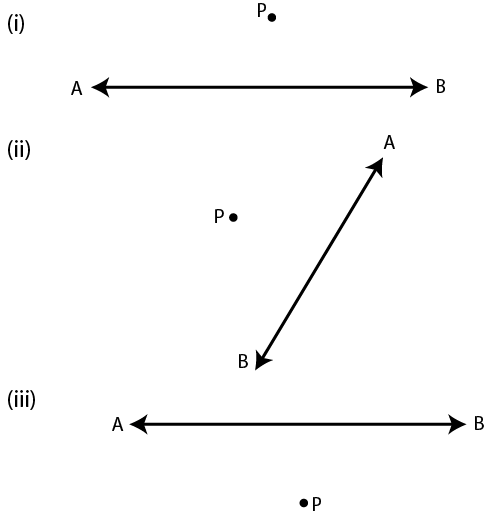Solution:

Steps of Construction:

1. From the point P construct a line segment meeting AB

2. Taking Q as centre and some suitable radius construct an arc CD.

3. Taking P as centre and same radius construct another arc which meets PQ at the point E.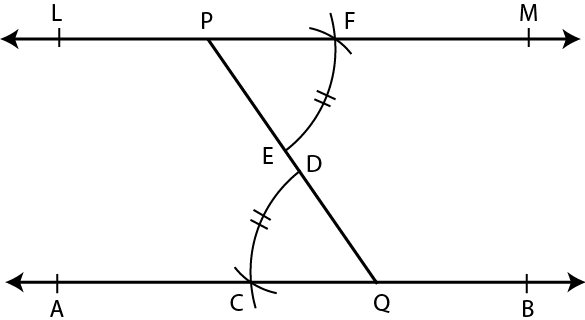4. Taking E as centre and radius equal to CD cut this arc at the point F.5. Now join PF and produce it to both sides to L and M.

Here the line LM is parallel to the given line AB.